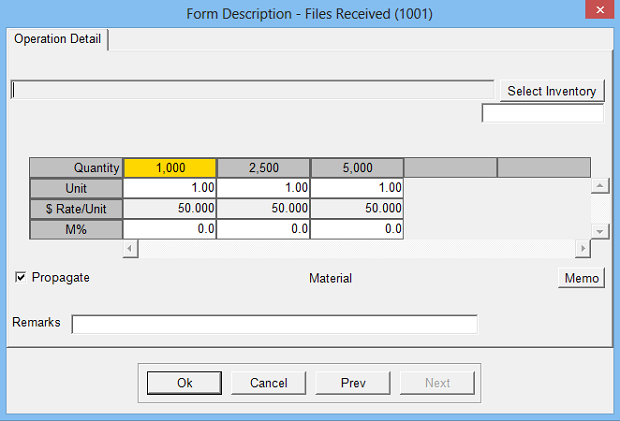Estimates material or labor costs with a fixed unit price where production (time) standards do not apply. A unit may be pieces, minutes, hours, etc.

Operation Setup Requirements:
1. Description
2. Rate: Cost per unit
3. Sell \$ use: Markup % (recommended), alternate sell rate or sell grid
4. Calc: 1
5. Sub Key: Labor or Material
6. Set units to: Option to default units to pre-assigned number, pages, sheets, form quantity, etc.
7. Rate Per M: Makes \$ Rate per thousand
8. Rate Override: Allows user to change unit rate on Estimate screen
9. JC Cost By Quantity: Job Control Cost uses Quantity x Rate (vs. Hours x Rate). Only available if subkey is labor.Estimate Specification Input Prompts:

1. Units (not editable if set to pages, sheets or form quantity)
2. Rate (can be changed only if rate override checked in definition)
3. J% or Markup %
4. Remarks
5. MemoCalculation:

Units x Rate = Cost \$

Cost \$ x Markup % = Markup \$

Cost \$ + Markup \$ = Sell \$